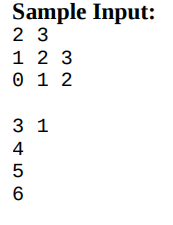1. Engineering
2. Computer Science
3. what is the c code to multiply two 2d matrix...

# Question: what is the c code to multiply two 2d matrix...

###### Question details

What is the C++ Code to multiply two 2d matrix stored as a double linked lists with four pointers(up, down, left, right)?

so image wize it would look like this###### Solution by an expert tutor